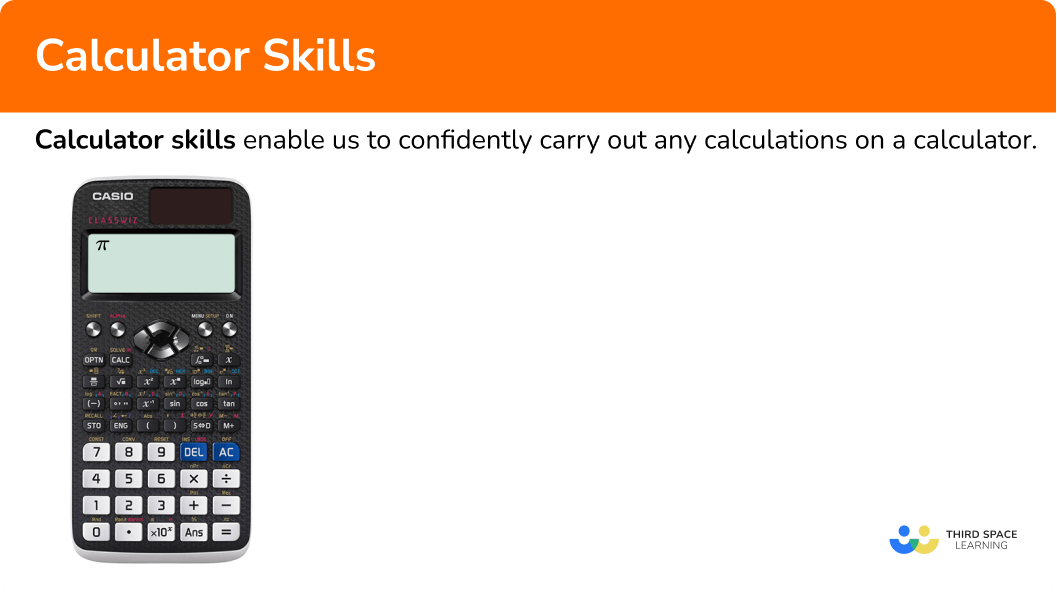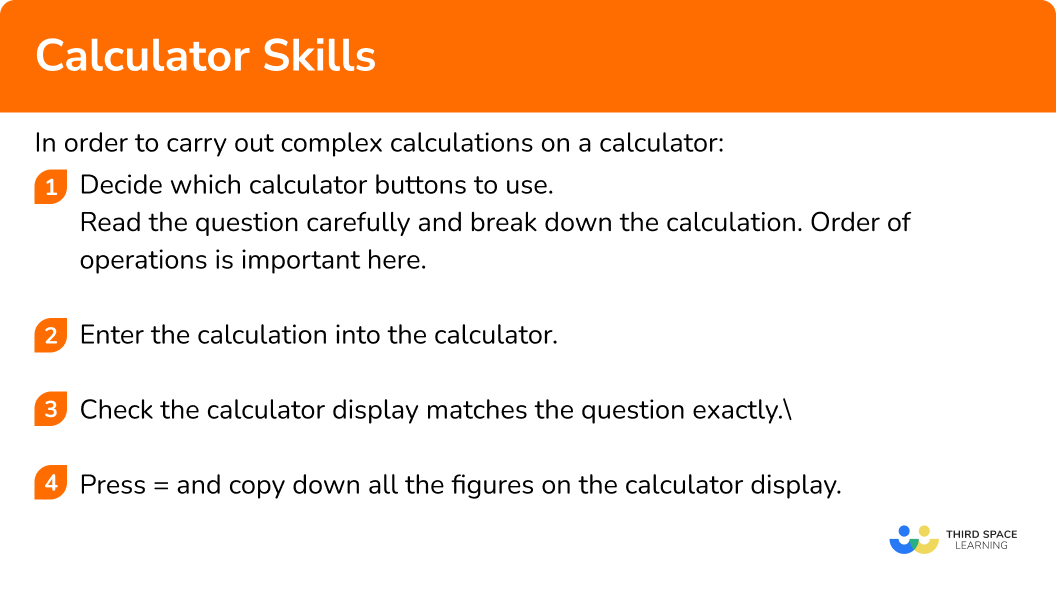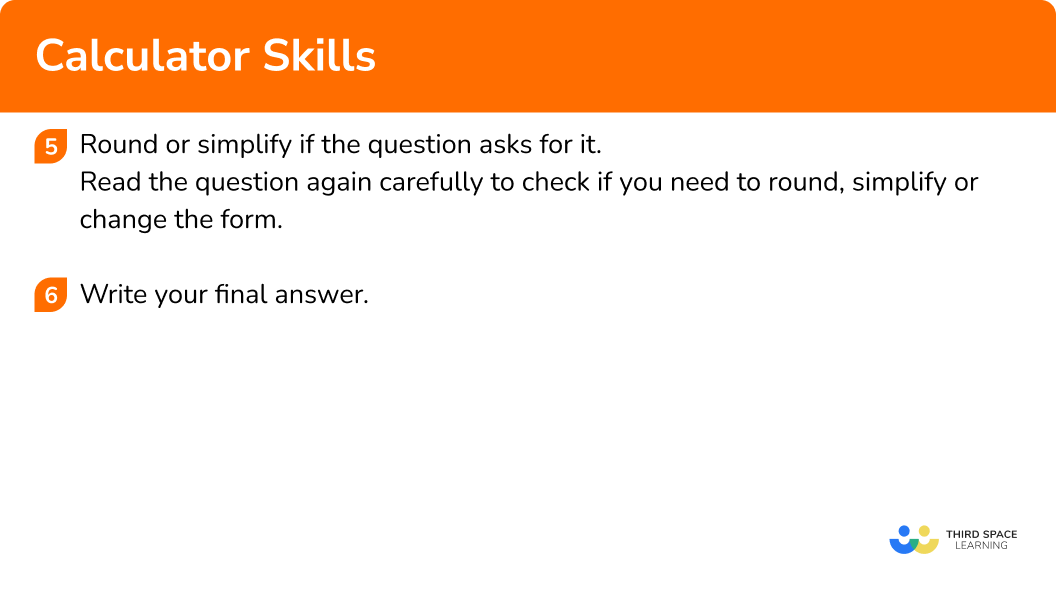GCSE Maths Number Arithmetic

Calculator Skills

# Calculator Skills

Here we will learn about calculator skills, including the buttons that help us navigate the calculator display with ease, functions that we need for basic calculations and how to complete more complex calculations to demonstrate calculator proficiency.

There are also calculator skills worksheets based on Edexcel, AQA and OCR exam questions, along with further guidance on where to go next if you’re still stuck.

## What are calculator skills?

Calculator skills enable us to confidently carry out any calculations on a calculator.

We need to know how to use several specific buttons to be proficient using a calculator for complex calculations.

• The toggle button switches an answer between its fraction and decimal form, root and decimal form, or π and decimal form.

• The shift button allows us to access the second functions printed in gold, for example those highlighted here.

• We can navigate back through our working on a calculator, to make changes or to check what we have entered, using the direction pad. We also need to use this button when entering calculations with fractions, roots and powers.

• The fraction button can be used to carry out calculations with fractions.

• We may have to enter complicated fractions into the calculator. To do this press the fraction button and enter the calculation on the top of the fraction, exactly as it is written in the question. Next use the directional pad to move to the bottom of the fraction and enter the calculation on the bottom of the fraction, exactly as it is written in the question.

• The x^2 and x^3 buttons can be used to square and cube numbers. We need to use brackets when dealing with negative numbers.

For example, if we want to square -4, we need to write (-4)^2

We can also raise numbers to any power using the \bf{x^∎} button. Again, we need to remember to use brackets for any negative numbers.

• We can calculate a square root on a calculator using the square root button. It is important to use the direction pad to move out of the square root box if we have more to add to the calculation before pressing the equals button.

• We can cube root a number using the cube root button. The cube root button is a second function button, in gold. We press the shift button to access the cube root function above the square root button. Again we must remember to use the direction pad to move out of the cube root box if we have more to add to the calculation before pressing the equals button.

We can also calculate any root of a number using the \sqrt[∎]{□} button.

• We can use the percentage button, % , to find percentages of amounts or include percentages in calculations.

For example, to find 40\% of 300 we would type 40\% \times 300.

• We might also need to include trigonometric functions in calculations, either to calculate a missing length or angle in a triangle using trigonometry, or as part of a calculation to demonstrate our calculator skills.

The calculator automatically opens brackets when you press the sin, cos, or tan buttons. We must close these brackets before we complete the calculation. We must always make sure that what we have entered on the calculator display matches the questions exactly.

### What are calculator skills?## How to use calculator skills

In order to carry out complex calculations on a calculator:

1. Decide which calculator buttons to use.
Read the question carefully and break down the calculation. Order of operations is important here.
2. Enter the calculation into the calculator.
3. Check the calculator display matches the question exactly.
4. Press ​​= and copy down all the figures on the calculator display.
5. Round or simplify if the question asks for it.
Read the question again carefully to check if you need to round, simplify or change the form.

### Explain how to use calculator skills### Explain how to use calculator skills## Related lessons on arithmetic

Calculator skills is part of our series of lessons to support revision on arithmetic. You may find it helpful to start with the main arithmetic lesson for a summary of what to expect, or use the step by step guides below for further detail on individual topics. Other lessons in this series include:

## Calculator skills examples

### Example 1: fraction calculation on a calculator

1. Decide which calculator buttons to use.

We will need to use the fraction button.

2Enter the calculation into the calculator.

On the calculator we press the buttons,

3Check the calculator display matches the question exactly.

4Press ​​= and copy down all the figures on the calculator display.

The answer to this calculation is displayed as \frac{7}{6}.

5Round or simplify if the question asks for it.

We need to give the answer as a mixed number. To do this we need to press shift and use the toggle button on the calculator to convert our answer into a mixed number.

The final answer to this question is then displayed on the calculator.

The final answer is 1 \frac{1}{6}.

### Example 2: complex fraction calculation on a calculator

Calculate \frac{2+\sqrt{2}}{1+\sqrt{2}}.

Decide which calculator buttons to use.

Enter the calculation into the calculator.

Check the calculator display matches the question exactly.

Press ​​= and copy down all the figures on the calculator display.

Round or simplify if the question asks for it.

### Example 3: cube number on a calculator

Evaluate 8^{3}.

Decide which calculator buttons to use.

Enter the calculation into the calculator.

Check the calculator display matches the question exactly.

Press ​​= and copy down all the figures on the calculator display.

Round or simplify if the question asks for it.

### Example 4: complex calculation on a calculator

Decide which calculator buttons to use.

Enter the calculation into the calculator.

Check the calculator display matches the question exactly.

Press ​​= and copy down all the figures on the calculator display.

Round or simplify if the question asks for it.

### Example 5: complex calculation on a calculator

Use your calculator to work out \frac{\sqrt{12.36-5.12}}{2.97^{2}}.

Write down all the figures on your calculator display.

Decide which calculator buttons to use.

Enter the calculation into the calculator.

Check the calculator display matches the question exactly.

Press ​​= and copy down all the figures on the calculator display.

Round or simplify if the question asks for it.

### Example 6: complex calculation on a calculator

Use your calculator to work out \sqrt{12^{2}+15^{2}-54 \operatorname{Cos}(60)} .

Decide which calculator buttons to use.

Enter the calculation into the calculator.

Check the calculator display matches the question exactly.

Press ​​= and copy down all the figures on the calculator display.

Round or simplify if the question asks for it.

### Common misconceptions

• Evaluating the square of a negative number incorrectly on a calculator

We must use brackets if we use a calculator to square a negative.

• Rounding

Pay close attention to how you should give your answer – what form it should be in and whether it should be rounded.

• Moving out of a function

If you need to do something else after a function, make sure you move out of that function. For example, if you need to calculate \sqrt{7.2+4.1}-3, a common error is to not move out of the square root and therefore type \sqrt{7.2+4.1-3}.

You should notice that this is different to the original calculation, where the 3 is not within the square root function.

• Brackets for trigonometric functions

Make sure you close the brackets at the end of a trigonometric function. For example, if you want to calculate \cos(30)+6, typing cos into the calculator automatically gives you the open bracket. If you don’t close the bracket after you have typed 30, your calculation will be incorrect.

\cos(30+6) is different to \cos(30)+6 .

### Practice calculator skills questions

1. Use your calculator to work out \frac{3.21+4.89}{5.62-1.89}.

2.22.172.182.20\frac{3.21+4.89}{5.62-1.89} = 2.171581769 = 2.17 \ (2dp)

\frac{29}{72}\frac{3}{-1}\frac{3}{72}\frac{9}{14}\frac{5}{8} – \frac{2}{9} = \frac{29}{72}

3.750.6441.251.88\frac{\sqrt{8.4+19.2}+6}{3^{2}}=1.250396691…

4. Use your calculator to work out the following \sqrt{(-3)^{8}}.

Write down all figures on the calculator display.

81-1093.54.326748711Math error\sqrt{(-3)^{8}} = 4.326748711

161-11621593\sin(40)+12.6^{2}=160.6883628…

6. Use your calculator to work out \sqrt{\frac{\tan(30)+6^{2}}{5tan(30)}}. Write down all the digits on your calculator display.

3.5596019183.6122176132.0950613430.8820714039\sqrt{\frac{\tan(30)+6^{2}}{5tan(30)}}=3.559601918

### Calculator skills GCSE questions

1. Work out \sqrt{\frac{2.5 \times \sin(43)}{8.2^{2}-50.5}}.

(2 marks)

0.3191419855…

(1)

0.319

(1)

2. Work out \sqrt{\frac{17+4^{2}}{7.3^{2}}}.

Write down all the figures on your calculator display.

(2 marks)

5.74(45626…) or 53.29 or 0.11 or 0.107 or 0.108

(1)

0.1077981356

(1)

3. Work out \sqrt{\frac{13.82}{4.06}}.

Write down all the figures on your calculator display.

(2 marks)

3.403(940887…) or 3.717(526059…) or 2.014(944168…)

(1)

1.844977205

(1)

## Learning checklist

You have now learned how to:

• Use the operational buttons to navigate calculations on a calculator
• Know and use important buttons on the calculator to support problem solving
• Carry out complex calculations using a calculator

## Still stuck?

Prepare your KS4 students for maths GCSEs success with Third Space Learning. Weekly online one to one GCSE maths revision lessons delivered by expert maths tutors.

Find out more about our GCSE maths tuition programme.